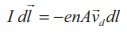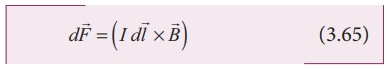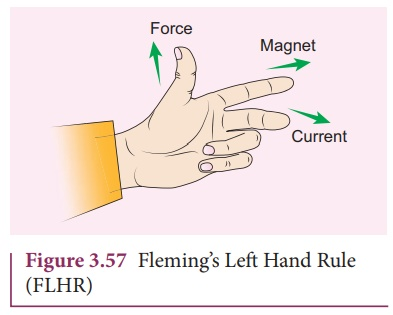Home | | Physics 12th Std | Force on a current carrying conductor placed in a magnetic field

# Force on a current carrying conductor placed in a magnetic field

Physics : Magnetism and Magnetic Effects of Electric Current: Lorentz Force: Force on a current carrying conductor placed in a magnetic field

## Force on a currentcarrying conductor placed in a magnetic fieldWhen a current carrying conductor is placed in a magnetic field, the force experienced by the wire is equal to the sum of Lorentz forces on the individual charge carriers in the wire. Consider a small segment of wire of length dl, with cross-sectional area A and current I as shown in Figure 3.56. The free electrons drift opposite to the direction of current. So the relation between current I and magnitude of drift velocity vd (Refer Unit 2) isIf the wire is kept in a magnetic field, then average force experienced by the charge (here, electron) in the wire isLet n be the number of free electrons per unit volume, thereforewhere N is the number of free electrons in the small element of volume V = A dl.

Hence Lorentz force on the wire of length dl is the product of the number of the electrons

(N = nA dl) and the force acting on an electron.The length dl is along the length of the wire and hence the current element in the wire isTherefore the force on the wire isThe force in a straight current carrying conducting wire of length l placed in a uniform magnetic field isIn magnitude,

F = BIl sinθ

(a) If the conductor is placed along the direction of the magnetic field, the angle between them is θ = 0º. Hence, the force experienced by the conductor is zero.

(b) If the conductor is placed perpendicular to the magnetic field, the angle between them is θ =90º Hence, the force experienced by the conductor is maximum, which is F = BIl.

### Fleming’s left hand rule (mnemonic)

When a current carrying conductor is placed in a magnetic field, the direction of the force experienced by it is given by Fleming’s Left Hand Rule (FLHR) as shown in Figure 3.57.Stretch forefinger, the middle finger and the thumb of the left hand such that they are in mutually perpendicular directions. If forefinger points the direction of magnetic field, the middle finger points the direction of the electric current, then thumb will point the direction of the force experienced by the conductor.

### EXAMPLE 3.27

A metallic rod of linear density is 0.25 kg m-1 is lying horizontally on a smooth inclined plane which makes an angle of 45º with the horizontal. The rod is not allowed to slide down by flowing a current through it when a magnetic field of strength 0.25 T is acting on it in the vertical direction. Calculate the electric current flowing in the rod to keep it stationary.### Solution

The linear density of the rod i.e., mass per unit length of the rod is 0.25 kg m-1

m/l = 0.25 kg m−1

Let I be the current flowing in the metallic rod. The direction of electric current is into the paper. The direction of magnetic force IBl is given by Fleming’s left hand rule.For equilibrium,

mg sin 45º = IBl cos 45 º

⇒ I = I/B m/l g tan 45 º

⇒ I = 9.8 A

So, we need to supply current of 9.8 A to keep the metallic rod stationary.

Tags : Lorentz Force | Physics , 12th Physics : Magnetism and Magnetic Effects of Electric Current
Study Material, Lecturing Notes, Assignment, Reference, Wiki description explanation, brief detail
12th Physics : Magnetism and Magnetic Effects of Electric Current : Force on a current carrying conductor placed in a magnetic field | Lorentz Force | Physics